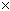SEARCH HOMEMath Central Quandaries & QueriesQuestion from Mary: Can you place these in order? 1/8 15/64 15/16 7/32Hi Mary.

When you use a common denominator (bottom number) for all the fractions, you can simply compare the numerators (top numbers). So convert the fractions to equivalent fractions that all have a common denominator, then compare them.

Here is an example.

Place in order from smallest to largest: 2/3, 7/9, 59/81, 22/27.

All the denominators go into 81 (327, 99, 811, 273).

2/3 = (227) / (327) = 54/81,

7/9 = (79) / (99) = 63/81,

59/81 = (591) / (811) = 59/81 and

22/27 = (223) / (273) = 66/81.

Putting these in order we have 54/81, 59/81, 63/81, 66/81.

This corresponds to 2/3, 59/81, 7/9, 22/27.

Hope this example helps you with your own fractions.
Stephen La Rocque.Math Central is supported by the University of Regina and The Pacific Institute for the Mathematical Sciences.